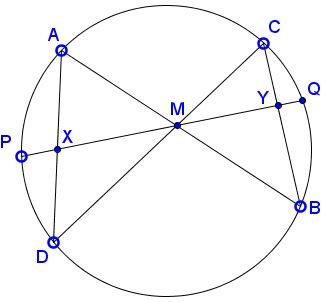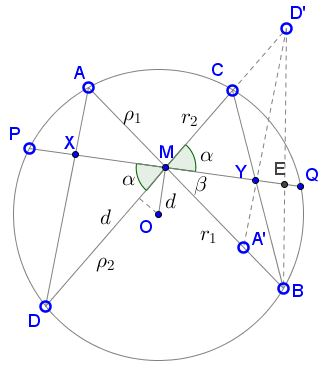# A Proof of the Butterfly Theorem Using Ceva�s Theorem

### Theorem (The Butterfly Theorem)

Through the midpoint $M\;$ of a chord $PQ\;$ of a circle, two other chords $AB\;$ and $CD\;$ are drawn. Chords $AD\;$ and $BC\;$ intersect $PQ\;$ at points $X\;$ and $Y,\;$ respectively.Then $M\;$ is also the midpoint of $XY.$

We introduce the points $A'\;$ and $D'\,$ that are the symmetric of $A\;$ and $D\;$ about $M,\;$ respectively. Hence, $MA' = MA\;$ and $MD' = MD.\;$ Next we connect the point $D'\;$ to $A'\;$ and $B,\;$ and call $E\;$ the intersection of $DB\;$ with the line through $P\;$ and $Q.\;$ Thus we have constructed triangle $MBD'\;$ with cevians $D'A',\;$ $ME,\;$ and $BC.\;$We show that the segment $DA\;$ cuts the chord $PQ\;$ at the same point $Y\;$ as $BC,\;$ i.e., that the three cevians are concurrent at $Y.\;$ This property will be proved by applying Ceva�s theorem to triangle $MBD'.\;$

### Lemma

In triangle $MBD,\;$ the cevians $DA,\;$ $ME,\;$ and $BC\;$ are concurrent at $Y.\;$

### Proof of Lemma

We set $MA'=MA=\rho_1,\;$ $MB=r_1,\;$ $MC=r_2\;$ and $MD'=MD=\rho_2.\;$ We observe that $\displaystyle\frac{BE}{ED'}=\frac{r_1\sin\beta}{\rho_2\sin\alpha},\;$ the ratio of respective distances of $B\;$ and $D'\;$ from the line $PQ.\;$ Moreover, $A'B=r_1-\rho_1,\;$ and $D'C=\rho_2-r_2.\;$ Now,

(1)

\displaystyle\begin{align} \frac{BE}{ED'}\cdot\frac{D'C}{CM}\cdot\frac{MA'}{A'B} &= \frac{r_1\sin\beta}{\rho_2\sin\alpha}\cdot\frac{\rho_2-r_2}{r_2}\cdot\frac{\rho_1}{r_1-\rho_1}\\ &=\frac{(\rho_2-r_2)\sin\beta}{(r_1-\rho_1)\sin\alpha}, \end{align}

since $\rho_1r_1=\rho_2r_2\;$ by the Intersecting Chords Theorem.

The differences appearing in (1) can be written in terms of the distance $d = OM\;$ of the circle center $O\;$ to the chord $PQ,\;$ and the angles $\alpha\;$ and $\beta.\;$ The above figure shows that the projection of $OM\;$ onto the chord $PQ\;$ has length $d\sin\alpha,\;$ so that we get $\displaystyle\rho_2=\frac{1}{2}CD+d\sin\alpha,\;$ and $\displaystyle r_2=\frac{1}{2}C-d\sin\alpha.\;$ Hence, $\rho_2-r_2=2d\sin\alpha.\;$ Similarly, we find $r_1-\rho_1=2d\sin\beta.$ Substituting these expressions into (1) we obtain

$\displaystyle\frac{BE}{ED'}\cdot\frac{D'C}{CM}\cdot\frac{MA'}{A'B}=1.$

By Ceva's Theorem, the cevians $D'A',\;$ $ME,\;$ and $BC\;$ are concurrent. The common point is clearly $Y.$

### Proof of Butterfly Theorem

We observe that triangle $MA'D'\;$ is congruent by construction to triangle $MAD,\;$ because two sides of the first $(MA',MD')\;$ are equal to two sides of the second $(MA,MD),\;$ and the included angles are equal. It follows that $\angle MD'Y = \angle MDX.\;$ Consequently, triangles $MD'Y\;$ and $MDX\;$ are also congruent, since they have equal two pairs of angles $(\angle MD'Y = \angle MDX,\;$ and $\angle YMD'= \angle XMD,\;$ vertical angles), as well as the included sides $(MD' = MD).\;$ This congruence implies that the corresponding sides $MY\;$ and $MX\;$ are equal. Therefore, $M\;$ is the midpoint of $XY\;$ and the butterfly theorem is proved.

### Remark

It is rather interesting to compare this proof to William Wallace's 1803 Statement.### Butterfly Theorem and Variants